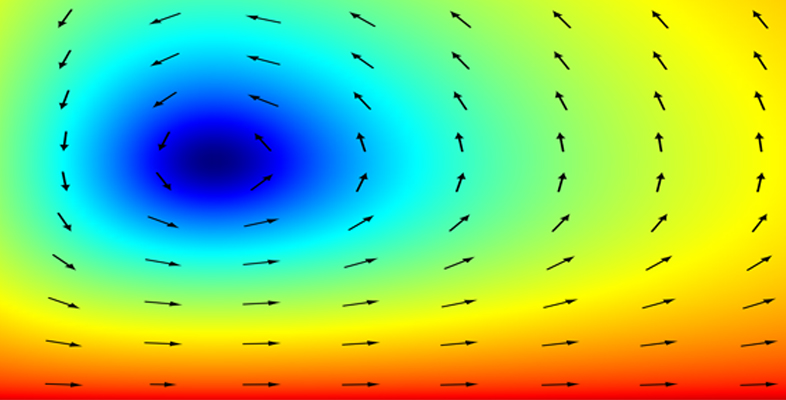Dynamics

Start this free course now. Just create an account and sign in. Enrol on the course to track your learning.

Free course

# Learning outcomes

After studying this course, you should be able to:

• understand and use basic terms for the description of the motion of particles, vector functions and the fundamental laws of Newtonian mechanics

• solve mechanics problems in one dimension that involve one or more of the forces of gravity, friction and air resistance

• understand the concept of terminal speed, and use it in solving mechanics problems in one dimension

• apply Newton’s second law in vector form to problems in more than one dimension

• solve problems relating to the motion of a projectile in the absence of air resistance.

MST210_1